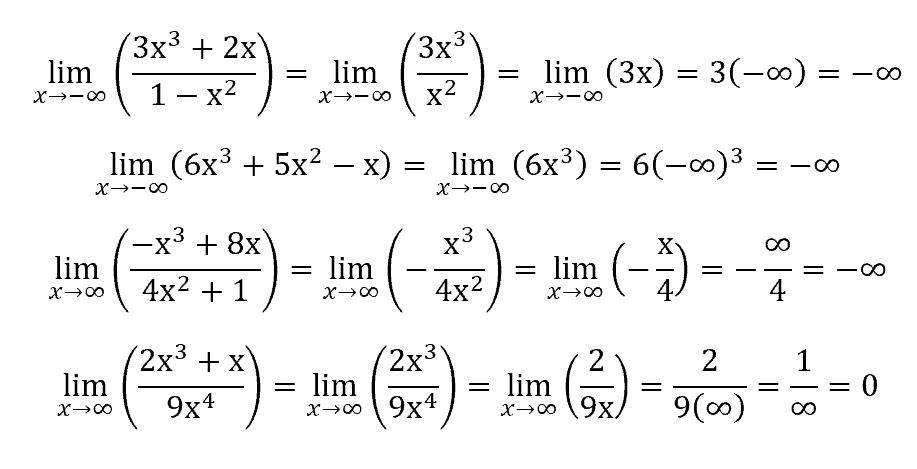# PCAT Quantitative Reasoning – Equivalent Limits

• by
• Oct 26, 2017
• PCAT Question of the Day

##### Explanation

D is correct. When evaluating limits approaching infinity, we must consider only the terms in the numerator and denominator with the highest degree. In other words, if you see a polynomial fraction, cancel out every term except for the term in the numerator with the largest exponent and the term in the denominator with the largest exponent. Then plug in the value that x approaches for every x and solve: